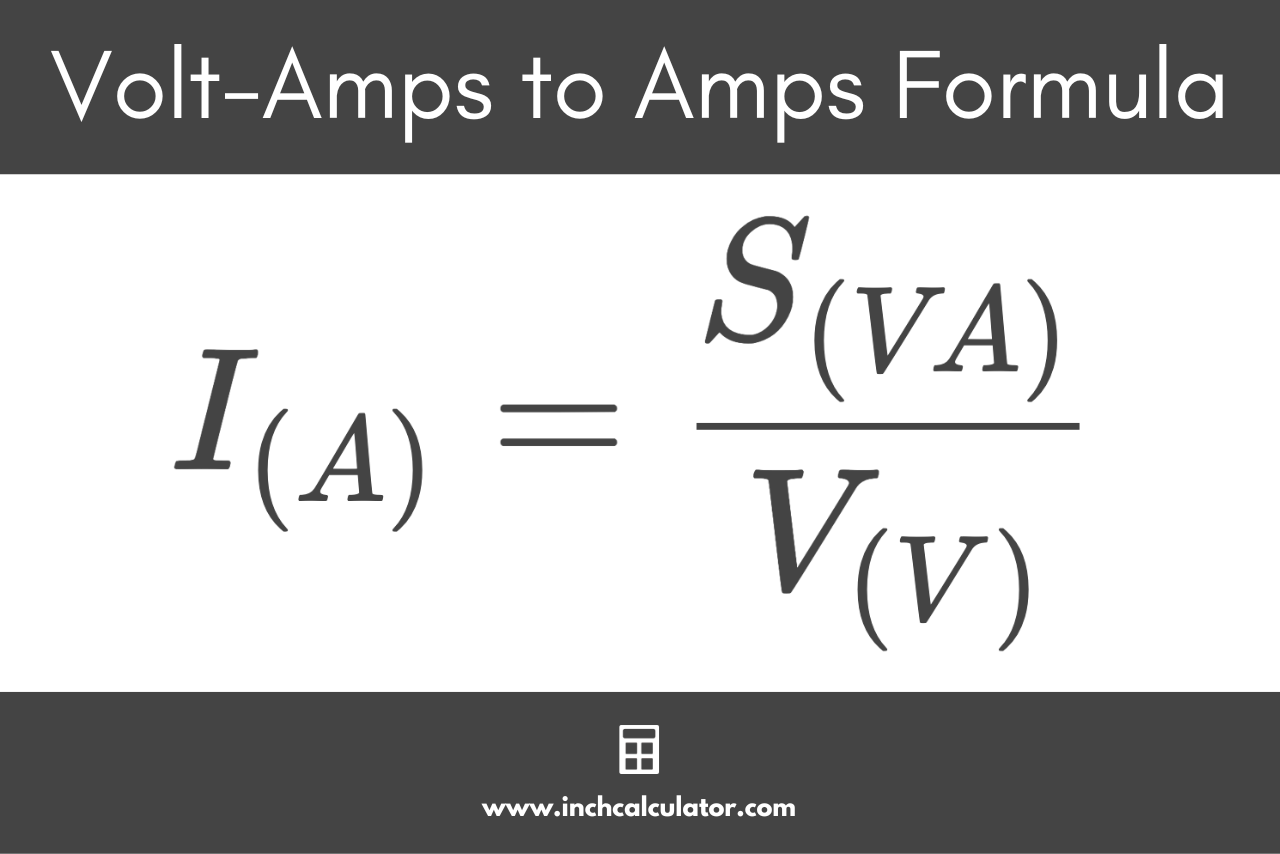# Volt-amps (VA) to Amps Conversion Calculator

Convert volt-amps to amps by entering the power and voltage below. The calculator supports single-phase and three-phase circuits.

volt-amps
volts

## Current:

Amps
Learn how we calculated this below

## How to Convert Volt-amperes to Amps

Before we can convert volt-amps to amps, we need to understand a little bit more about what these measurements are. A volt-ampere (VA) is a measure of the apparent electrical power, while an amp, or ampere, (A) is a measure of electrical current.

Thus, to convert between them, we need to use the Watt’s Law power formula, which states that the electrical power is equal to the voltage times the current.
Power = Voltage × Current

Using the power formula as the starting point, by rearranging the formula, you can convert the apparent power in volt-amps to current in amps:

I(A) = S(VA) / V(V)

Thus, the current I in amps is equal to the apparent power S in volt-amps divided by the voltage V. Converting volt-amps to amps is as simple as that.For example, let’s find the amps for a single-phase electrical circuit with 1,800 volt-amps of power at 120 volts.

I(A) = 1,800 VA / 120 V
I(A) = 15 A

So, a circuit with 1,800 volt-amps of apparent power at 120 volts has a current rating of 15 amps.

You can use our amps to volt-amps calculator to reverse this conversion.

### How to Convert Volt-amps to Amps for Three-Phase Circuits

The volt-amps to amps conversion is a bit different for a three-phase electrical circuit. To calculate, use the modified three-phase formula.

I(A) = S(VA) / √3 × VL-L(V)

For a three-phase electrical circuit, the current I in amps is equal to the apparent power S in volt-amps divided by the square root of three times the line to line voltage V.

In this formula, voltage is the line-to-line voltage of the circuit.

For example, let’s find the amps for a three-phase electrical circuit with 33,255 volt-amps of power at 480 volts.

I(A) = 33,255 VA / √3 × 480 V
I(A) = 33,255 VA / 831.38 V
I(A) = 40 A

We can see that a circuit with 33,255 VA of apparent power at 480 V will have a current rating of 40 A.